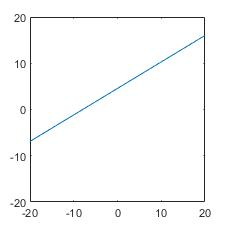# Output of CNN used for regression converges to mean of training data

Hi everyone!
I used a CNN to do regression on a trainings set to estimate the parameters of a line (mx+b). For this purpose I generated Images with Matlab which plots one line (consisting of randomly initialized values for m and b in the range of [-10,10]) per picture.

While training, my outputs converge to 0 for m and b since this is the mean value of both my inputs.

sample image:The CNN used looks like this:

``````    def __init__(self):
self.layer1 = nn.Sequential(
nn.Conv2d(1, 6, kernel_size=2, stride=1),
nn.ReLU(),
nn.MaxPool2d(kernel_size=2, stride=2))
self.layer2 = nn.Sequential(
nn.Conv2d(6, 16, kernel_size=3, stride=1),
nn.ReLU(),
nn.MaxPool2d(kernel_size=2, stride=2))
self.drop_out = nn.Dropout()
self.fc1 = nn.Linear(16 * 55 * 55, 120)
self.fc2 = nn.Linear(120, 84)
self.fc3 = nn.Linear(84, 2)

def forward(self, x):
out = self.layer1(x)
out = self.layer2(out)
out = out.reshape(out.size(0), -1)
out = self.fc1(out)
out = self.fc2(out)
out = self.fc3(out)
return out
``````

Is the CNN used for this task correct? How should I improve my model to do better in regression?